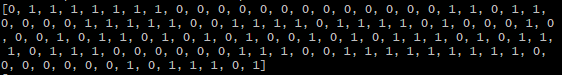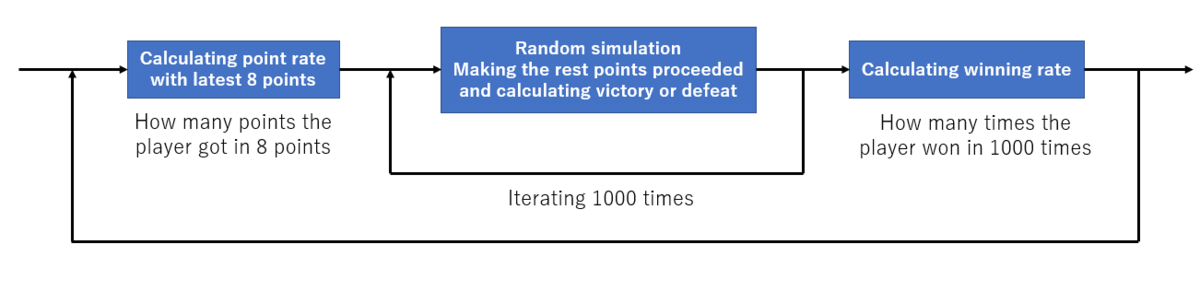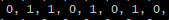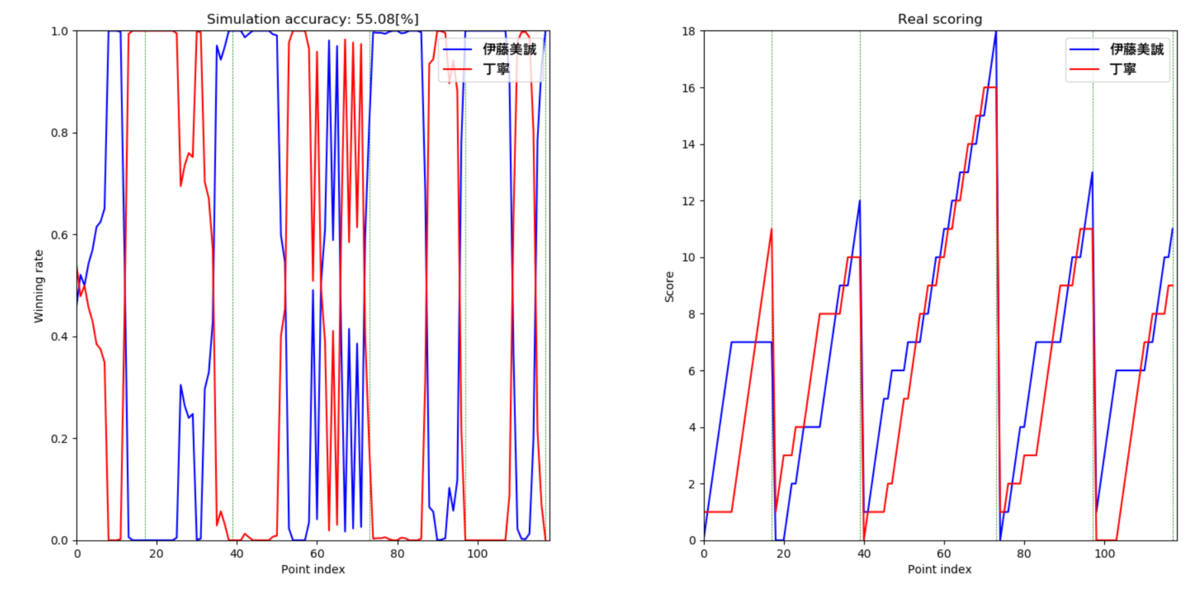# Momentum shift analysis of Table tennis game ~Winning Rate Simulation~

## Introduction

I have started Table tennis game analysis wrote the following article before.
www.eureka-moments-blog.com
My goal of this analysis is visualizing a momentum shift of game and predicting come-from-ahead loss. In this article, I tried to create a winning rate simulation and introduce about it in detail.

## Reference

I created the simulation by referring to the following articles.

## Input data

• 2019 China OP, Ito Mima vs Ding Ning.
• Real result is 4-1, Ito won.
• This simulation uses a binary(0 or 1) array as follow.• Player 1 is defined as 1 and player 2 is defined as 0. In the above array, 1 means that player 1 got a point and 0 means that player 2 got a point.

## Source code

```# -*- coding: utf-8 -*-

import tkinter as tk
import tkinter.filedialog as tkfd
import pandas as pd
import numpy as np
import matplotlib.pyplot as plt
from matplotlib.font_manager import FontProperties

class TableTennisSimulator:

def __init__(self):
self.df_org        = None
self.player_name_1 = None
self.player_name_2 = None
self.game_match_num = 0
self.calc_interval_point = 0
self.win_rate_1 = np.array([])
self.win_rate_2 = np.array([])

def set_game_match_num(self):
game_match_1 = self.df_org['player1Game'].values[-1]
game_match_2 = self.df_org['player2Game'].values[-1]
if game_match_1 > game_match_2:
self.game_match_num = game_match_1
else:
self.game_match_num = game_match_2

def set_calc_interval_point(self):
calc_interval_point_str = input('Please input calculation interval point: ')
self.calc_interval_point = int(calc_interval_point_str)

fType = [('CSV', '*.csv')]
csv_path = tkfd.askopenfilename(title='Select csv files',
filetypes=fType)
if not csv_path:
print('Select csv file')
else:
self.df_org = pd.read_csv(csv_path, encoding='shift-jis')
self.player_name_1 = self.df_org['player1'].values
self.player_name_2 = self.df_org['player2'].values
self.set_game_match_num()
self.set_calc_interval_point()

def sigmoid_func(self, x):
y = 1.0 / (1.0 + np.exp(-x))
return y

def calc_score_rate(self, sc_sum_1, sc_sum_2):
x = sc_sum_1 / (sc_sum_1 + sc_sum_2)
y = self.sigmoid_func(x-0.5)
return x

def random_single_game(self, sc1, sc2, g1, g2, sc_rt, gm):
score_1 = sc1
score_2 = sc2
game_1  = g1
game_2  = g2
# count score and game
for i in range(1000):
random = np.random.rand()
score_1 = score_1 + 1 if random < sc_rt else score_1
score_2 = score_2 + 1 if random > sc_rt else score_2
if score_1 >= 11 or score_2 >= 11:
if abs(score_1 - score_2) >= 2:
game_1 = game_1 + 1 if score_1 > score_2 else game_1
game_2 = game_2 + 1 if score_2 > score_1 else game_2
score_1 = 0
score_2 = 0
if game_1 >= gm:
return 1
if game_2 >= gm:
return 0
return

def random_roop(self, sc1, sc2, g1, g2, sc_rt, gm):
game_result_array = np.array([])
for i in range(1000):
game_result = self.random_single_game(sc1, sc2, g1, g2, sc_rt, gm)
game_result_array = np.append(game_result_array, game_result)
return game_result_array.sum()/1000

def simulate_game(self):
self.score_1    = self.df_org['player1Score'].values
self.score_2    = self.df_org['player2Score'].values
self.game_1     = self.df_org['player1Game'].values
self.game_2     = self.df_org['player2Game'].values
self.get_player = self.df_org['getPointPlayer'].values
self.get_player_01 = []
self.get_player_01 = [gp*0 if gp == 2 else gp for gp in self.get_player]
self.get_player_01_sim = []
self.game_bound_index  = []
prev_game_1 = self.game_1
prev_game_2 = self.game_2
for i, (sc1, sc2, g1, g2) in enumerate(zip(self.score_1, self.score_2, self.game_1, self.game_2)):
if i >= self.calc_interval_point:
# score rate
sc_sum_1  = sum(self.get_player_01[i-self.calc_interval_point:i])
sc_sum_2  = self.calc_interval_point - sc_sum_1
sc_rate_1 = self.calc_score_rate(sc_sum_1, sc_sum_2)
else:
sc_rate_1 = 0.5
# game changed boundary index
if g1 != prev_game_1 or g2 != prev_game_2:
self.game_bound_index.append(i)
prev_game_1 = g1
prev_game_2 = g2
# winning rate
win_rate_1 = self.random_roop(sc1, sc2, g1, g2, sc_rate_1, self.game_match_num)
win_rate_2 = 1 - win_rate_1
self.win_rate_1 = np.hstack((self.win_rate_1, [win_rate_1]))
self.win_rate_2 = np.hstack((self.win_rate_2, [win_rate_2]))
if win_rate_1 > win_rate_2:
self.get_player_01_sim.append(1)
else:
self.get_player_01_sim.append(0)
self.calculate_accuracy()

def calculate_accuracy(self):
total_point = len(self.get_player_01)
same_point  = 0
self.accuracy = 0
for i, (real, sim) in enumerate(zip(self.get_player_01, self.get_player_01_sim)):
if real == sim:
same_point += 1
self.accuracy = (same_point / total_point) * 100

def show_sim_result(self):
fp = FontProperties(fname=r'C:\Windows\Fonts\YuGothB.ttc', size=12)
if max(self.score_1) > max(self.score_2):
max_score = max(self.score_1)
else:
max_score = max(self.score_2)
fig = plt.figure()
ax_wr.plot(range(len(self.get_player_01)), self.win_rate_1, c='blue', label=self.player_name_1)
ax_wr.plot(range(len(self.get_player_01)), self.win_rate_2, c='red', label=self.player_name_2)
ax_wr.vlines(self.game_bound_index, 0.0, 1.0, linestyle='dashed', linewidth=0.5, colors='green')
ax_wr.set_xlim(0, len(self.get_player_01))
ax_wr.set_ylim(0.0, 1.0)
ax_wr.set_xlabel('Point index')
ax_wr.set_ylabel('Winning rate')
ax_wr.set_title('Simulation accuracy: {0:.2f}[%]'.format(self.accuracy))
ax_wr.legend(prop=fp, loc='upper right')
ax_sc.plot(range(len(self.get_player_01)), self.score_1, c='blue', label=self.player_name_1)
ax_sc.plot(range(len(self.get_player_01)), self.score_2, c='red', label=self.player_name_2)
ax_sc.vlines(self.game_bound_index, 0.0, max_score, linestyle='dashed', linewidth=0.5, colors='green')
ax_sc.set_xlim(0, len(self.get_player_01))
ax_sc.set_ylim(0.0, max_score)
ax_sc.set_xlabel('Point index')
ax_sc.set_ylabel('Score')
ax_sc.set_title('Real scoring')
ax_sc.legend(prop=fp, loc='upper right')
plt.tight_layout()
plt.show()

if __name__ == "__main__":

sim = TableTennisSimulator()

root = tk.Tk()
root.withdraw()

sim.simulate_game()

sim.show_sim_result()
```

## Usage

1. Execution command is, "python TableTennisSimulator.py"
2. After the above command was executed, a window to select and open a csv file is opend.
3. You need to select a csv file. The sample files are located at my GitHub repository.
4. After the csv file was opened, you will be asked "Please input calculation interval point: ".
5. This interval point is used as a parameter for point rate calculation.
6. After this parameter was input, the simulation will be executed and displayed a result graph.

## Simulation algorithm

### Overview### Calculating point rate

At each point, a point rate of each player is calculated with the latest 8 points.
For example, the following 8 points mean that player 1 got 4 points and the other player got 4 points too. In this case, a probability player 1 got a point is 50%(4/8) and a probability player 2 got a point is 50%(4/8).### 1000 times random simulation

Assuming that each player get a point with the above calculated probability, a winning player of the game will be predicted. This prediction is simulated 1000 times and a winning rate of player is calculated. For example, if player 1 won 200 times and player 2 won 800 times, the winning rate of player 1 would be 20%. This simulation is iterated at each point.

## Simulation result

The following 2 graphs are the simulation result. The left side graph is the calculated winning rate transition. The right side one is a real scoring transition.About Ito Mima(blue line), when she got a points continuously in the right side graph, her winning rate is increasing at the left side graph. It looks that this simulation is accurate but the simulated points and real points do not match completely. This matching rate is 55.08%. It is not accurate. And then, the calculated winning rate sometimes were 100% or 0%. I think that the winning rate 100% or 0% is impossible.

## Next action

I'm gonna try improving this simulation performance from now on. Especially, how to calculate a point rate at each point need to be modified.